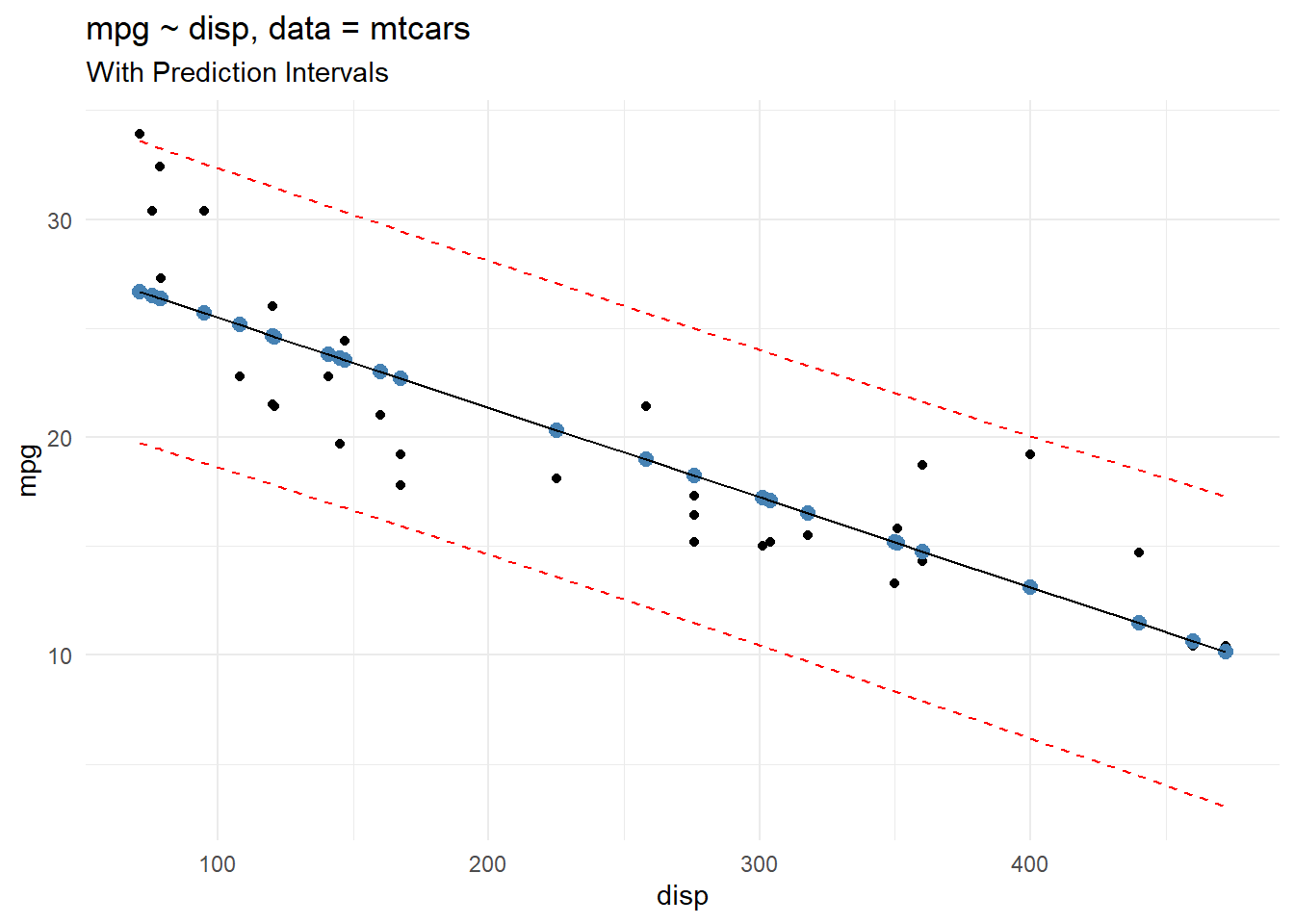# Unlocking the Power of Prediction Intervals in R: A Practical Guide

rtip
Author

Steven P. Sanderson II, MPH

Published

November 13, 2023

# Introduction

Prediction intervals are a powerful tool for understanding the uncertainty of your predictions. They allow you to specify a range of values within which you are confident that the true value will fall. This can be useful for many tasks, such as setting realistic goals, making informed decisions, and communicating your findings to others.

In this blog post, we will show you how to create a prediction interval in R using the `mtcars` dataset. The `mtcars` dataset is a built-in dataset in R that contains information about fuel economy, weight, displacement, and other characteristics of 32 cars.

# Creating a Prediction Interval

To create a prediction interval in R, we can use the `predict()` function. The `predict()` function takes a fitted model and a new dataset as input and returns the predicted values for the new dataset.

We can also use the `predict()` function to calculate prediction intervals. To do this, we need to specify the `interval` argument. The `interval` argument can take two values: `confidence` and `prediction`.

A confidence interval is the range of values within which we are confident that the true mean of the population will fall. A prediction interval is the range of values within which we are confident that the true value of a new observation will fall.

To create a prediction interval for the `mpg` variable in the `mtcars` dataset, we can use the following code:

``````# Fit a linear model
model <- lm(mpg ~ disp, data = mtcars)

# Create a prediction interval
prediction_intervals <- predict(
model,
newdata = mtcars,
interval = "prediction",
level = 0.95
)

# Print the prediction interval
``````                       fit       lwr      upr
Mazda RX4         23.00544 16.227868 29.78300
Mazda RX4 Wag     23.00544 16.227868 29.78300
Datsun 710        25.14862 18.302683 31.99456
Hornet 4 Drive    18.96635 12.217933 25.71477
Valiant           20.32645 13.582915 27.06999``````

The prediction interval shows that we are 95% confident that the true `mpg` value for a new car with a given displacement will fall within the range specified by the `lwr` and `upr` columns.

# Visualize

First lets bind the data together with `cbind()`

``````full_res <- cbind(mtcars, prediction_intervals)

``````                   mpg cyl disp  hp drat    wt  qsec vs am gear carb      fit
Mazda RX4         21.0   6  160 110 3.90 2.620 16.46  0  1    4    4 23.00544
Mazda RX4 Wag     21.0   6  160 110 3.90 2.875 17.02  0  1    4    4 23.00544
Datsun 710        22.8   4  108  93 3.85 2.320 18.61  1  1    4    1 25.14862
Hornet 4 Drive    21.4   6  258 110 3.08 3.215 19.44  1  0    3    1 18.96635
Hornet Sportabout 18.7   8  360 175 3.15 3.440 17.02  0  0    3    2 14.76241
Valiant           18.1   6  225 105 2.76 3.460 20.22  1  0    3    1 20.32645
lwr      upr
Mazda RX4         16.227868 29.78300
Mazda RX4 Wag     16.227868 29.78300
Datsun 710        18.302683 31.99456
Hornet 4 Drive    12.217933 25.71477
Valiant           13.582915 27.06999``````

Now let’s plot the actual, the fitted and the prediction confidence bands.

``````library(ggplot2)

full_res |>
ggplot(aes(x = disp, y = mpg)) +
geom_point() +
geom_point(aes(y = fit), col = "steelblue", size = 2.5) +
geom_line(aes(y = fit)) +
geom_line(aes(y = lwr), linetype = "dashed", col = "red") +
geom_line(aes(y = upr), linetype = "dashed", col = "red") +
theme_minimal() +
labs(
title = "mpg ~ disp, data = mtcars",
subtitle = "With Prediction Intervals"
)``````Above we are capturing the prediction interval which gives us the uncertainty around a single point, whereas the confidence interval gives us the uncertainty around the mean predicted values. This means that the prediction interval will always be wider than the confidence interval for the same value.

# Trying It Out Yourself

Now it’s your turn to try out creating a prediction interval in R. Here are some ideas:

• Try creating a prediction interval for a different variable in the `mtcars` dataset, such as `wt` or `hp`.
• Try creating a prediction interval for a variable in a different dataset.
• Try creating a prediction interval for a more complex model, such as a multiple linear regression model or a logistic regression model.

# Conclusion

Creating prediction intervals in R is a straightforward process. By using the `predict()` function, you can easily calculate prediction intervals for any fitted model and any new dataset. This can be a valuable tool for understanding the uncertainty of your predictions and making more informed decisions.